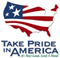# Generalized Estimates from Streamflow Data of Annual and Seasonal Ground-Water-Recharge Rates for Drainage Basins in New Hampshire

### Scientific Investigations Report 2004-5019

The body of the report is available in PDF format (7.63 MB)

The cover of report is available in PDF format (1.42 MB)

ERRATA SHEET in PDF format ( 57KB)

Abstract

This report presents regression equations to estimate generalized annual and seasonal ground-water-recharge rates in drainage basins in New Hampshire. The ultimate source of water for a ground-water withdrawal is aquifer recharge from a combination of precipitation on the aquifer, ground-water flow from upland basin areas, and infiltration from streambeds to the aquifer. An assessment of ground-water availability in a basin requires that recharge rates be estimated under `normal' conditions and under assumed drought conditions.

Recharge equations were developed by analyzing streamflow, basin characteristics, and precipitation at 55 unregulated continuous record stream-gaging stations in New Hampshire and in adjacent states. In the initial step, streamflow records were analyzed to estimate a series of annual and seasonal ground-water-recharge components of streamflow in each drainage basin evaluated in this study. Regression equations were then developed relating the series of annual and seasonal ground-water-recharge values to the corresponding series of annual and seasonal precipitation values as determined at the centroid of each drainage basin. This resulted in one equation for each of the 55 basins for each of the four seasonal periods and the annual period, or a total of 275 regression equations. Average annual and seasonal precipitation data for 1961-90 were then used to compute a set of normalized ground-water-recharge values that reflected the long-term average annual and seasonal variations (normalized) and mean recharge characteristics of each drainage basin. Ordinary-least-squares regression was applied in the process of selecting 10 out of 93 possible basin and climatic characteristics for further testing in the development of the equations for computing the generalized estimate of annual and seasonal ground-water recharge based on the set of normalized recharge values. Generalized-least-squares regression was used for the final parameter estimation and error evaluation. The following basin and climatic characteristics were found to be statistically significant predictors for at least one of the dependent variables: average annual, summer, and spring precipitation as determined at U.S. Geological Survey stream-gaging stations; average annual basin-centroid precipitation; average mean annual basin temperature; average minimum winter basin temperature; percent coniferous forest in a basin; percent mixed coniferous and deciduous forest in a basin; average fall basin-centroid precipitation; and average annual snowcover. These 10 basin and climatic characteristics were selected because they were statistically significant based on several statistical parameters that evaluated which combination of characteristics contributed the most to the predictive accuracy of the regression-equation models. A geographic information system is required to measure the values of the predictor variables for the equations developed in the study.

The average annual normalized ground-water recharge was 21.0 in. This value was determined by generalized-least-squares (GLS) regression analysis for all of the basins used in the normalized ground-water recharge analysis for precipitation from 1961-90. The average winter (January 1-March 15) ground-water recharge was 4.3 in., average spring (March 16-May 31) ground-water recharge was 9.0 in., average summer (June 1-October 31) ground-water recharge was 4.0 in., and average fall (November 1-December 31) ground-water recharge was 3.6 in. Normalized ground-water recharge ranged annually from 12.3 to 31.8 in., for winter from 2.30 to 7.82 in., for spring from 5.16 to 13.7 in., for summer from 1.45 to 10.2 in., and for fall from 2.21 to 6.06 in.

Abstract

Introduction

Purpose and Scope

Previous Studies

Description of Study Area

Database Development

Streamflow Data

Precipitation Data

Basin-Characteristics Data

Methods of Analysis

Ordinary-Least-Squares Regression Equations

Generalized-Least-Squares Regression Equations

Regional Regression for Normalized Annual and Seasonal Recharge Using Generalized-Least-Squares Regression

Estimates of Annual and Seasonal Ground-Water-Recharge Rates

Estimation of Ground-Water-Recharge Rates from Stream-Gaging Station Data

Normalization of Ground-Water-Recharge Rates Using Precipitation Data

Generalized Estimate of Drainage-Basin Ground-Water-Recharge Rates Using Climatic and Basin Characteristics Data

Ground-Water-Recharge Regression Equations

Physical Basis for the Independent Variables in the Regression Equations

Uncertainties in Determining Ground-Water Recharge

Limitations on the Use of Regression Equations

Summary and Conclusions

Acknowledgments

Selected References

Appendix 1. Basin and Climate Characteristics Tested for Significance in the Ground-Water Recharge Regression Analysis

For further information, contact

Brian Mrazik,

District Chief

USGS

361 Commerce Way

Pembroke, NH 03275

dc_nh@usgs.gov

or

Robert Flynn, rflynn@usgs.gov

 AccessibilityFOIAPrivacyPolicies and Notices U.S. Department of the Interior, U.S. Geological Survey Persistent URL: Page Contact Information: Contact USGS Last modified: Thursday, January 10 2013, 06:28:25 PM